# Dual space (functional analysis)

In mathematics, particularly in the branch of functional analysis, a dual space refers to the space of all continuous linear functionals on a real or complex Banach space. The dual space of a Banach space is again a Banach space when it is endowed with the operator norm. If X is a Banach space then its dual space is often denoted by X'.

## Definition

Let X be a Banach space over a field F which is real or complex, then the dual space X' of$X$ is the vector space over F of all continuous linear functionals$f:\,X \rightarrow \,F$ when F is endowed with the standard Euclidean topology.

The dual space$X'$ is again a Banach space when it is endowed with the operator norm. Here the operator norm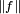$\|f\|$ of an element$f \,\in\, X'$ is defined as: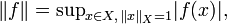$\|f\|=\mathop{\sup}_{x \in X,\,\|x\|_X=1} |f(x)|,$

where$\|\cdot\|_X$ denotes the norm on X.

## The bidual space and reflexive Banach spaces

Since X' is also a Banach space, one may define the dual space of the dual, often referred to as a bidual space of X and denoted as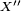$X''$. There are special Banach spaces X where one has that$X''$ coincides with X (i.e.,$X''\,=\, X$), in which case one says that X is a reflexive Banach space (to be more precise,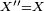$X''=X$ here means that every element of$X''$ corresponds to some element of$X$ as described in the next section).

An important class of reflexive Banach spaces are the Hilbert spaces, i.e., every Hilbert space is a reflexive Banach space. This follows from an important result known as the Riesz representation theorem.

## Dual pairings

If X is a Banach space then one may define a bilinear form or pairing$\langle x,x' \rangle$ between any element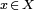$x \,\in\, X$ and any element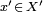$x' \,\in\, X'$ defined by$\langle x,x' \rangle =x'(x).$

Notice that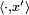$\langle \cdot,x'\rangle$ defines a continuous linear functional on X for each$x' \,\in\, X'$, while$\langle x,\cdot\rangle$ defines a continuous linear functional on$X'$ for each$x \,\in\, X$. It is often convenient to also express$x(x')= \langle x,x' \rangle =x'(x),$

i.e., a continuous linear functional f on$X'$ is identified as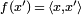$f(x')\,=\,\langle x,x' \rangle$ for a unique element$x \,\in\, X$. For a reflexive Banach space such bilinear pairings determine all continuous linear functionals on X and$X'$ since it holds that every functional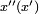$x''(x')$ with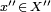$x'' \,\in\, X''$ can be expressed as$x''(x')\,=\,x'(x)$ for some unique element$x \,\in\, X$.

Dual pairings play an important role in many branches of mathematics, for example in the duality theory of convex optimization.